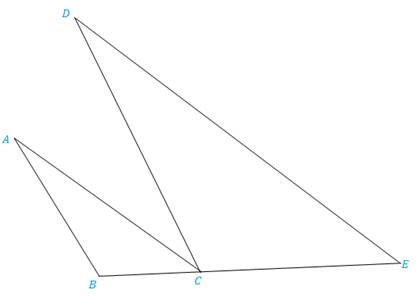Chapter 5.3, Problem 24EElementary Geometry For College St...

7th Edition
Alexander + 2 others
ISBN: 9781337614085

Solutions

Chapter
SectionElementary Geometry For College St...

7th Edition
Alexander + 2 others
ISBN: 9781337614085
Textbook Problem

In Exercises 17 to 24, complete each proof.Given: A B ¯ ∥ D C ¯ , A C ¯ ∥ D E ¯ Prove: A B D C = B C C EPROOF Statements Reasons 1. A B ¯ ∥ D C ¯ 2.3. ?4. ∠ A C B ≅ ∠ E 5. Δ A C B ∼ Δ D E C 6. ? 1. ?2. If 2 || lines are cut by a transversal, corresponding ∠ s are ≅ .3. Given4. ?5. ?6. ?

To determine

To prove:

The statement ABDC=BCCE if the sides AB and DC are parallel, AB¯DC¯ and the sides AC and DE are parallel, AC¯DE¯.

Explanation

Definition:

AA:

If two angles of one triangle are congruent to two angles of another triangle, then the triangles are similar.

CSSTP:

Corresponding sides of similar triangles are proportional.

Description:

Given that RS¯UV¯.

The given figure is shown below.

Figure

From the given figure, it is observed that the sides AB and DC are parallel, AB¯DC¯.

If two parallel lines are cut by a transversal, then the alternate interior angles are congruent.

Therefore, BACD.

From the given figure, it is also observed that the sides AC and DE are parallel, AC¯DE¯.

If two parallel lines are cut by a transversal, then the alternate interior angles are congruent.

Therefore, ACBE.

The above mentioned AA definition, the two triangles ACB and DEC are similar since the two angles of one triangle are congruent to two angles of another triangle. Hence, ΔACBΔDEC.

From the definition of CSSTP, corresponding sides of similar triangles are proportional

Still sussing out bartleby?

Check out a sample textbook solution.

See a sample solution

The Solution to Your Study Problems

Bartleby provides explanations to thousands of textbook problems written by our experts, many with advanced degrees!

Get Started

Evaluate the expression sin Exercises 116. 22212424

Finite Mathematics and Applied Calculus (MindTap Course List)

Evaluate in problems 1-4. Write all the answers without using exponents. 1. (a) ...

Mathematical Applications for the Management, Life, and Social Sciences

Convert from degrees to radians. 5. 900

Single Variable Calculus: Early Transcendentals, Volume I